# Using a Voltage Divider - Why don't I got the output voltage that I calculated?

#### FelixB

Joined Dec 26, 2022
33
Hello everyone,
Today I wanted to build a parking assistance for a old car, but I stumbled upon a problem:
Using a HC-SR04(5V) with a Raspberry Pi Pico(3.3V) I have a problem:
The HC-SR04 outputs in 5V, which I don't wanna use to burn my Pico. So I builded my first Voltage Divider to drop 5V to 3.3V.
I found out that I need 1030Ω and 2000Ω resistorsto get the required output voltage. But after using a voltmeter to find out if I have the right output voltage I saw that I only get 2.45V.
Why do I get such a voltage drop?

Here are my calculations: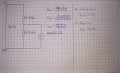And here is my full schematic: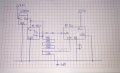#### bertus

Joined Apr 5, 2008
22,233
Hello,

The output voltage will be lower as the GP1 input will have an input impedance that is parallel with the R2 of your schematic.
A buffer circuit would eliminate the load from the GP1 input.
An opamp as voltage follower would do as buffer.

Bertus

#### MrChips

Joined Oct 2, 2009
29,858
You can use the circuit as is or you can adjust your resistors to get the desired values.
I would no worry about perfect trimming, i.e. I would omit the 10Ω resistors.
Try 1kΩ + 2.2kΩ resistors.

#### FelixB

Joined Dec 26, 2022
33
I also got a AMS1117-3.3V voltage regulator laying around. Could I just use this instead of the voltage divider?

#### kerry_wiegand

Joined Sep 16, 2023
1
Your full schematic shows the output from the 5V regulator is directly connected to the ECHO pin on the sensor, which would lead to excessive current being sunk by the ECHO pin. I suggest disconnecting the sensor and measuring the output of the 5V regulator AND measuring the output of your divider with the regulator connected to it. If the regulator output is close to 5V your divider output should be close to 3.3V.

You should be aware that the output of the ECHO pin output may be less than 5V, since the datasheet only specifies "TTL compatible output". If you have an oscilloscope you could check the output amplitude of the ECHO pin (without anything connected to it). A volt meter will give you an average of the voltage.

The output impedance of the sensor output and the input impedance of the PICO GPIO pin will also factor into the actual voltage at the divider output. Ideally the sensor output impedance is zero and the GPIO input impedance is infinity but that won't be the case in reality. I would expect the GPIO input impedance would be fairly high as long as the voltage is lower than the I/O voltage supply (3.3V).

#### FelixB

Joined Dec 26, 2022
33
Oh I forgot to mention that: I only connected the Vcc of the HC-SR04 with the echo, because I wanted to measure it and simulate a output of the echo pin. Because it was a test I only drew that with a pencil.

#### BobTPH

Joined Jun 5, 2013
8,120
So is the voltage divider connected to the output or to Vcc? It is still not clear.

FYI, the output will not be 5V.

#### Ian0

Joined Aug 7, 2020
8,948
Hello everyone,
Today I wanted to build a parking assistance for a old car, but I stumbled upon a problem:
Using a HC-SR04(5V) with a Raspberry Pi Pico(3.3V) I have a problem:
The HC-SR04 outputs in 5V, which I don't wanna use to burn my Pico. So I builded my first Voltage Divider to drop 5V to 3.3V.
I found out that I need 1030Ω and 2000Ω resistorsto get the required output voltage. But after using a voltmeter to find out if I have the right output voltage I saw that I only get 2.45V.
Why do I get such a voltage drop?

Here are my calculations:
View attachment 302939

And here is my full schematic:
View attachment 302940
What voltage do you get across the 2k resistor if GP1 is not connected to it? Does the voltage change when you make and break the connection to GP1? Do you have GP1 set as an input? Does GP1 have a built-in pull-down resistor?

#### crutschow

Joined Mar 14, 2008
33,365
One important fact about using a divider to get a reduced voltage, is that the output looks like a voltage source in series with the parallel resistance value of the two resistors forming the divider.
Thus any load on the voltage will reduce the voltage by the load current times that parallel resistance.

#### Jon Chandler

Joined Jun 12, 2008
853
Illustrating what @crutschow said, look at the following pictures. The first shows the basic voltage divider, neglecting the load resistance.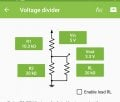The second picture shows the output voltage when the load resistance is considered – 20k is a reasonable value for a microcontroller input.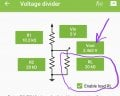"R2" effectively becomes the parallel value of R2 and RL.

#### WBahn

Joined Mar 31, 2012
29,519
Hello everyone,
Today I wanted to build a parking assistance for a old car, but I stumbled upon a problem:
Using a HC-SR04(5V) with a Raspberry Pi Pico(3.3V) I have a problem:
The HC-SR04 outputs in 5V, which I don't wanna use to burn my Pico. So I builded my first Voltage Divider to drop 5V to 3.3V.
I found out that I need 1030Ω and 2000Ω resistorsto get the required output voltage. But after using a voltmeter to find out if I have the right output voltage I saw that I only get 2.45V.
Why do I get such a voltage drop?

Here are my calculations:
View attachment 302939

And here is my full schematic:
View attachment 302940
Are you assuming that the output of the Echo pin is actually 5V?

The data sheet merely says that it is a TTL output. As is so common with modules like this, they are woefully incomplete.

So let's take them at their word and assume that the output conforms to the TTL specifications.

Here is the classic TTL output: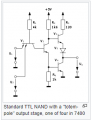Notice that there is not only a 130 Ω resistor in the HI output path, but also a base-emitter drop and a diode drop. Thus, even with almost no output current, you are unlikely to see more than about 3.6 V at the output. The data sheet (such as the TI7400 data sheet) says that the output is typically 3.4 V when sourcing the maximum output load current, which is only 0.4 mA.

Your resistor chain is about 3 kΩ, which means you are asking it to supply more than a full milliamp, and are thus already exceeding the TTL spec even before you connect it to the R-Pi.